# NCERT Solutions for Class 10 Maths Chapter 7:Coordinate Geometry Exercise 7.1

In this page we have NCERT Solutions for Class 10 Maths Chapter 7:Coordinate Geometry for Exercise 7.1 on pages 161 and 162. Hope you like them and do not forget to like , social share and comment at the end of the page.

# Coordinate Geometry Exercise 7.1

Question 1.
Find the distance between the following pairs of points :
(i) (2, 3), (4, 1)
(ii) (-5, 7), (-1, 3)
(iii) (a,b), (-a,-b)
Solution
Distance between the points AB is given by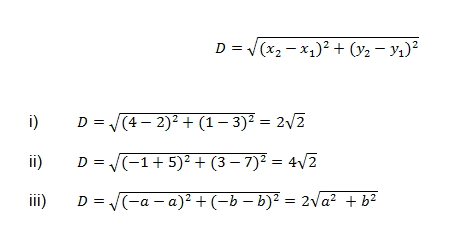Question 2.
Find the distance between the points (0, 0) and (36, 15). Can you now find the distance between the two towns A and B .
Solution
Let P(0,0) and Q(36,15) be the given points.
Distance between the points PQ is given b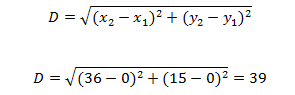The position of town A and B can be represented as points P and Q respectively,So distance between town will be 39 km

Question 3.
Determine if the points (1, 5), (2, 3) and (-2, -11) are collinear.
Solution
Lets us denote point by P(1,5) , Q(2,3) and R(-2,-11)
If the points are not collinear, then we should be able to form the triangle
Lets us find the length of PQ, QR and PR by distance formula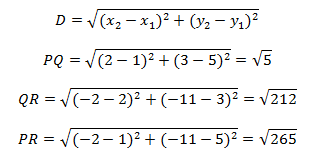Clearly None of these is true
PQ+QR=PR
PR+PQ=QR
PQ=QR+PR
Hence they are not collinear

Question 4.
Check whether (5, -2), (6, 4) and (7, -2) are the vertices of an isosceles triangle
Solution
Lets us denote point by P(5,-2) , Q(6,4) and R(7,-2)
Lets us find the length of PQ, QR and PR by distance formula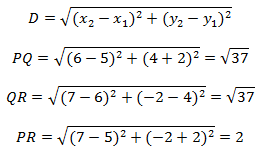Now PQ =QR ,so it is an isosceles triangle

Question 5.
In a classroom, 4 friends are seated at the points A, B, C and D as shown below. Champaand Chameli walk into the class and after observing for a few minutes Champa asks Chameli,
Solution
As per the figure given,The coordinates of the points A,B,C and D are (3,4), (6,7) ,(9,4) and (6,1)
Lets us find the length of AB, BC ,CD and AD by distance formula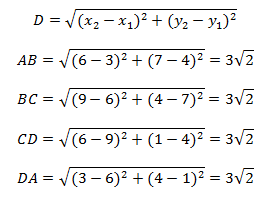So all the sides are equal. But we cannot still say that it is square as rhombus has all the sides equal also.
Now we know that a square has both the diagonal equal also,So lets us calculate the diagonal’s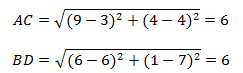Hence AC=BD
So, it is a square.
So, champa is correct
“Don’t you think ABCD is a square?” Chameli disagrees.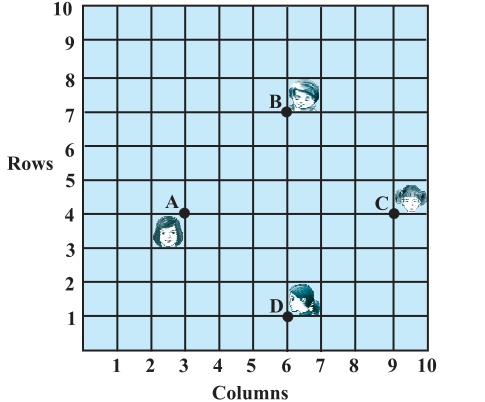Using distance formula, find which of them is correct.

Question 6.
Name the type of quadrilateral formed, if any, by the following points, and give reasons for your answer:
(i) (-1, -2), (1, 0), (-1, 2), (-3, 0)
(ii) (-3, 5), (3, 1), (0, 3), (-1, -4)
iii) (4,5) ,(7,6), (4,3) (1,2)
Solution
In these type of problem, we need to find of length of each segment, then check with the properties of different type of quadrilateral
i.e for points A,B,C and D
Line segments are AB,BC,AC,CD,AD and BD
We need to find the length of each of these
(i) (-1, -2), (1, 0), (-1, 2), (-3, 0)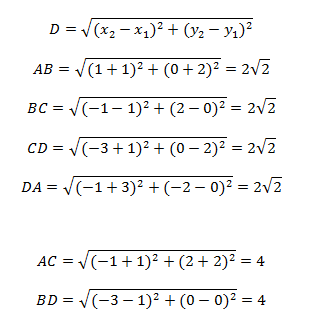Here the side AB,BC,CD and AD are equal and diagonal AC and BD are equal. So this is a square
(ii) (-3, 5), (3, 1), (0, 3), (-1, -4)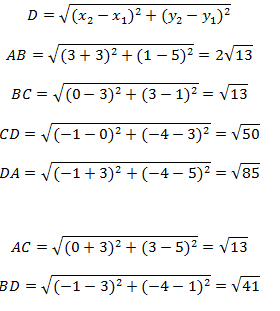Now here AC+BC=AB
So, that means ABC are collinear points.
So, it is not a quadrilateral infact
(iii) (4,5) ,(7,6), (4,3) (1,2)Now AB=CD and BC=DA
Now it could be rectangle or parallelogram
But diagonal AC is not equal diagonal BD
So, It is a parallelogram

Question 7.
Find the point on the x-axis which is equidistant from (2, –5) and (–2, 9).
Solution
Since the point lies on X axis, the point should be of the form (a,0)
Now (a,0) is equidistant from both the given points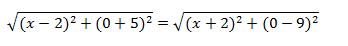Squaring both the sides
(x-2)2 + (0+5)2 =(x+2)2 + (0-9)2
Solving it we get
x=-7

Question 8.
Find the values of y for which the distance between the points P(2, – 3) and Q(10, y) is 10 units
Solution
According to the question
PQ=10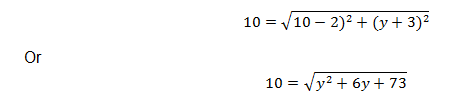Squaring both the sides
y2 +6y-27=0
(y+9)(y-3)=0
So y=-9 or 3

Question 9.
If Q(0, 1) is equidistant from P(5, –3) and R(x, 6), find the values of x. Also find the distances QR and PR.
Solution
Now
QP=QR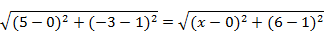Squaring both the sides
25+16=x2 +25
x= -4 or 4
So point R is either (4,6) or (-4,6)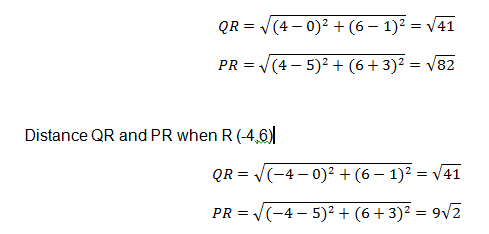Question 10.
Find a relation between x and y such that the point (x, y) is equidistant from the point
(3, 6) and (– 3, 4).
Solution
Let the point P(x,y) is equidistant from the point Q( 3,6) and R(-3,4)
PQ=PR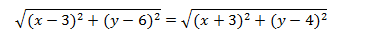Squaring both the sides
x2 -6x+9 +y2-12y +36=x2 +6x+9 +y2 -8y+16
-12x-4y+20=0
Or
3x+y-5=0 ( dividing by -4)

## Summary

1. NCERT Solutions for Class 10 Maths Chapter 7:Coordinate Geometry Exercise 7.1 has been prepared by Expert with utmost care. If you find any mistake.Please do provide feedback on mail. You can download the solutions as PDF in the below Link also
2. This chapter 7 has total 3 Exercise 7.1 ,7.2 and 7.3. This is the First exercise in the chapter.You can explore previous exercise of this chapter by clicking the link belowGo back to Class 10 Main Page using below links

### Practice Question

Question 1 What is $1 - \sqrt {3}$ ?
A) Non terminating repeating
B) Non terminating non repeating
C) Terminating
D) None of the above
Question 2 The volume of the largest right circular cone that can be cut out from a cube of edge 4.2 cm is?
A) 19.4 cm3
B) 12 cm3
C) 78.6 cm3
D) 58.2 cm3
Question 3 The sum of the first three terms of an AP is 33. If the product of the first and the third term exceeds the second term by 29, the AP is ?
A) 2 ,21,11
B) 1,10,19
C) -1 ,8,17
D) 2 ,11,20